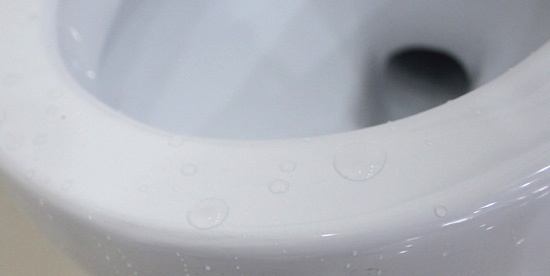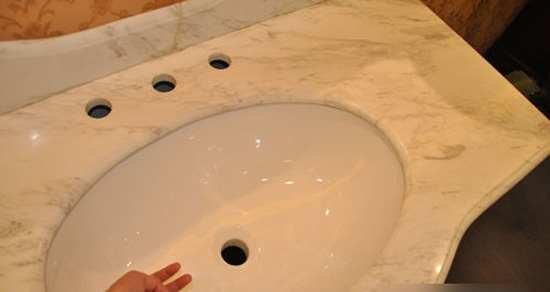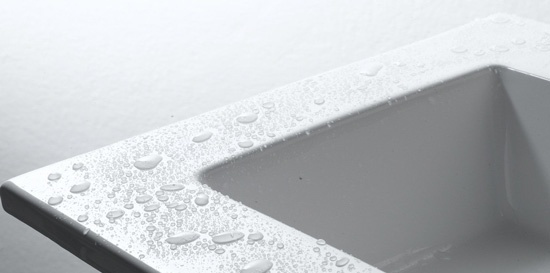|

# 卫浴市场的“庐山真面目” 你被骗过吗?

有过装修经验的人不难发现，陶瓷卫浴市场鱼目混珠、以次充好现象严重，价格也是参差不齐，经常出现同一品牌、同一型号卫浴产品，价格不同的现象，一不小心，就会掉进商家设计的“陷阱”。本期的陷阱实录，小编就为大家揭开陶瓷卫浴市场的“庐山真面目”，让我们一起来看看商家在销售过程中都会设下哪些“圈套”吧！一、案例回顾

胡先生在某知名卫浴品牌专卖店花了1万余元订购了一整套卫浴产品，商家送货时胡先生只清点了产品数量并未全部打开验货。

次日，待商家安装时发现其中一面盆上有裂纹，与商家交涉退货未果。商家称胡先生已在送货单上“经验货数量和外观完好无误”一栏下签字，对面盆有裂纹商家不再担责，只能维修，不能退换货。而胡先生坚持认为，对已包装好的卫浴产品往往只有等安装时才能发现问题，收货时签字不代表认可商品安装后可以正常使用，商家难咎其责。

二、陶瓷卫浴市场六大陷阱

陷阱一：虚高价格

价格陷阱，在现如今的卫浴市场中可谓屡见不鲜。同一件品质或品牌的商品，在不同的商家手中，价格可能会出现三四成甚至一倍的差距。而许多消费者也常常会在不知不觉中就消费了高价产品，在经济上蒙受损失。

在价格陷阱之中，许多不良商家为吸引顾客，最常使用的便是“明降暗涨”这一手段。有些看似大力的优惠，很有可能并没有实际上的让利。对于这种情况，消费者切不可有急切心理，先以随意的态度和商家讨价还价，试探商家口风，是否还存在隐瞒的价格底线。一般来说，越是正规的大品牌，这类隐藏价格的可能性越小，但即使是大品牌也可能有极个别的经销商违背道德约束，所以多一份心思对于消费者来说绝非坏事。

陷阱二：以次充好

消费者在选择陶瓷卫浴时，通常会注意到陶瓷卫浴的外包装上有标明等级，但是很少会知道这等级也有文章可做。现在陶瓷卫浴生产厂家对于等级的划分并不规范，有些厂家一级品就是优等品，因此，买陶瓷卫浴时最好问清商品品牌厂家的等级划分。但通常，优等品是最好的，一级品、二级品次之。有些不良商家，会将一级品、二级品冒充优等品出售，售价自然高了很多。

要避免这类以次充好的消费陷阱，消费者就应该在选购产品的过程中尽量去认准正规、具备知名度和良好口碑的品牌。

陷阱三：偷天换日

虽然消费者在店中看中品牌产品，却不料有些不良商家采取了调包行为，待送到消费者家中时却是同样花色款式的其他品牌陶瓷卫浴。如果消费者有异议，则退回产品，若消费者没有看出破绽，则乘机赚取差价。

对于消费者来说，要避免这些问题，就必须在消费合约之中仔细填写清楚，将产品的品牌、型号全部标明。只有这样，才能让消费者在遇上消费纠纷的过程中，有据可依。

陷阱四：“伪绿色”泛滥

环保，是如今许多消费者选购卫浴产品的重要参考依据之一。具备环保性能的卫浴产品，能够极大的节省卫浴间的耗水量。但也正是抓住消费者这一消费心理，许多无良商家常常会夸大吹嘘，即使毫无环保卖点的产品，也会被冠以各种节水、安全、无污染等特色来欺骗消费者。

在这样的情况下，消费者要秉承“耳听为虚眼见为实”的理念。仔细的去查看卫浴产品的介绍书，其中关于排水率，五金配件的重金属含量等，都必须亲眼确认，才能够彻底放心的进行消费。

陷阱五：不明码标价

有些陶瓷卫浴店里，并不是所有的商品都有标价。不良商家往往只标出其中一种价格较低的商品，待消费者查问其他商品时，乘机哄抬价格，让消费者在不知不觉中陷入商家设计的价格陷阱中。

陷阱六：以假乱真

市场上，名牌陶瓷卫浴无论在花色还是款式上，都是引领时尚潮流，吸引不少消费者的眼光。因此，有些商家利用消费者追求品牌的心理，制作出一些仿品牌款式的陶瓷卫浴，售价却只有名牌的一半或三分之一，有些消费者因贪图便宜买下了这种仿制品，却得不到品牌的服务及质量保证。

三、陶瓷卫浴外观质量的四个辨别方法：一看：消费者可选择在较强光线下，从侧面仔细观察卫浴产品表面的反光，表面没有或少有砂眼和麻点的为好。亮度指标高的产品采用了高质量的釉面材料和非常好的施釉工艺，对光的反射性好，从而视觉效果好。二摸：可用手在表面轻轻摩擦，感觉非常平整细腻的为好。还可以摸到背面，感觉有“沙沙”的细微摩擦感为好。三听：可用手敲击陶瓷表面，一般好的陶瓷材质被敲击发出的声音是比较清脆的。四比较：主要是在考察吸水率，吸水率越低的越好。陶瓷产品对水有一定的吸附渗透能力，水如果被吸进陶瓷，会产生一定的膨胀，容易使陶瓷表面的釉面因膨胀而龟裂。尤其对于坐便器，如果吸水率高，则很容易将水中的脏物和异味吸入陶瓷，时间一长就会产生无法去除的异味。

`声明：本文由入驻焦点开放平台的作者撰写，除焦点官方账号外，观点仅代表作者本人，不代表焦点立场错误信息举报电话： 400-099-0099，邮箱：jubao@vip.sohu.com，或点此进行意见反馈，或点此进行举报投诉。`A B C D E F G H J K L M N P Q R S T W X Y Z
A - B - C - D - E
• A
• 鞍山
• 安庆
• 安阳
• 安顺
• 安康
• 澳门
• B
• 北京
• 保定
• 包头
• 巴彦淖尔
• 本溪
• 蚌埠
• 亳州
• 滨州
• 北海
• 百色
• 巴中
• 毕节
• 保山
• 宝鸡
• 白银
• 巴州
• C
• 承德
• 沧州
• 长治
• 赤峰
• 朝阳
• 长春
• 常州
• 滁州
• 池州
• 长沙
• 常德
• 郴州
• 潮州
• 崇左
• 重庆
• 成都
• 楚雄
• 昌都
• 慈溪
• 常熟
• D
• 大同
• 大连
• 丹东
• 大庆
• 东营
• 德州
• 东莞
• 德阳
• 达州
• 大理
• 德宏
• 定西
• 儋州
• 东平
• E
• 鄂尔多斯
• 鄂州
• 恩施
F - G - H - I - J
• F
• 抚顺
• 阜新
• 阜阳
• 福州
• 抚州
• 佛山
• 防城港
• G
• 赣州
• 广州
• 桂林
• 贵港
• 广元
• 广安
• 贵阳
• 固原
• H
• 邯郸
• 衡水
• 呼和浩特
• 呼伦贝尔
• 葫芦岛
• 哈尔滨
• 黑河
• 淮安
• 杭州
• 湖州
• 合肥
• 淮南
• 淮北
• 黄山
• 菏泽
• 鹤壁
• 黄石
• 黄冈
• 衡阳
• 怀化
• 惠州
• 河源
• 贺州
• 河池
• 海口
• 红河
• 汉中
• 海东
• 怀来
• I
• J
• 晋中
• 锦州
• 吉林
• 鸡西
• 佳木斯
• 嘉兴
• 金华
• 景德镇
• 九江
• 吉安
• 济南
• 济宁
• 焦作
• 荆门
• 荆州
• 江门
• 揭阳
• 金昌
• 酒泉
• 嘉峪关
K - L - M - N - P
• K
• 开封
• 昆明
• 昆山
• L
• 廊坊
• 临汾
• 辽阳
• 连云港
• 丽水
• 六安
• 龙岩
• 莱芜
• 临沂
• 聊城
• 洛阳
• 漯河
• 娄底
• 柳州
• 来宾
• 泸州
• 乐山
• 六盘水
• 丽江
• 临沧
• 拉萨
• 林芝
• 兰州
• 陇南
• M
• 牡丹江
• 马鞍山
• 茂名
• 梅州
• 绵阳
• 眉山
• N
• 南京
• 南通
• 宁波
• 南平
• 宁德
• 南昌
• 南阳
• 南宁
• 内江
• 南充
• P
• 盘锦
• 莆田
• 平顶山
• 濮阳
• 攀枝花
• 普洱
• 平凉
Q - R - S - T - W
• Q
• 秦皇岛
• 齐齐哈尔
• 衢州
• 泉州
• 青岛
• 清远
• 钦州
• 黔南
• 曲靖
• 庆阳
• R
• 日照
• 日喀则
• S
• 石家庄
• 沈阳
• 双鸭山
• 绥化
• 上海
• 苏州
• 宿迁
• 绍兴
• 宿州
• 三明
• 上饶
• 三门峡
• 商丘
• 十堰
• 随州
• 邵阳
• 韶关
• 深圳
• 汕头
• 汕尾
• 三亚
• 三沙
• 遂宁
• 山南
• 商洛
• 石嘴山
• T
• 天津
• 唐山
• 太原
• 通辽
• 铁岭
• 泰州
• 台州
• 铜陵
• 泰安
• 铜仁
• 铜川
• 天水
• 天门
• W
• 乌海
• 乌兰察布
• 无锡
• 温州
• 芜湖
• 潍坊
• 威海
• 武汉
• 梧州
• 渭南
• 武威
• 吴忠
• 乌鲁木齐
X - Y - Z
• X
• 邢台
• 徐州
• 宣城
• 厦门
• 新乡
• 许昌
• 信阳
• 襄阳
• 孝感
• 咸宁
• 湘潭
• 湘西
• 西双版纳
• 西安
• 咸阳
• 西宁
• 仙桃
• 西昌
• Y
• 运城
• 营口
• 盐城
• 扬州
• 鹰潭
• 宜春
• 烟台
• 宜昌
• 岳阳
• 益阳
• 永州
• 阳江
• 云浮
• 玉林
• 宜宾
• 雅安
• 玉溪
• 延安
• 榆林
• 银川
• Z
• 张家口
• 镇江
• 舟山
• 漳州
• 淄博
• 枣庄
• 郑州
• 周口
• 驻马店
• 株洲
• 张家界
• 珠海
• 湛江
• 肇庆
• 中山
• 自贡
• 资阳
• 遵义
• 昭通
• 张掖
• 中卫

1室1厅1厨1卫1阳台

1
2
3
4
5

0
1
2

1

1

0
1
2
3报名成功，资料已提交审核A B C D E F G H J K L M N P Q R S T W X Y Z
A - B - C - D - E
• A
• 鞍山
• 安庆
• 安阳
• 安顺
• 安康
• 澳门
• B
• 北京
• 保定
• 包头
• 巴彦淖尔
• 本溪
• 蚌埠
• 亳州
• 滨州
• 北海
• 百色
• 巴中
• 毕节
• 保山
• 宝鸡
• 白银
• 巴州
• C
• 承德
• 沧州
• 长治
• 赤峰
• 朝阳
• 长春
• 常州
• 滁州
• 池州
• 长沙
• 常德
• 郴州
• 潮州
• 崇左
• 重庆
• 成都
• 楚雄
• 昌都
• 慈溪
• 常熟
• D
• 大同
• 大连
• 丹东
• 大庆
• 东营
• 德州
• 东莞
• 德阳
• 达州
• 大理
• 德宏
• 定西
• 儋州
• 东平
• E
• 鄂尔多斯
• 鄂州
• 恩施
F - G - H - I - J
• F
• 抚顺
• 阜新
• 阜阳
• 福州
• 抚州
• 佛山
• 防城港
• G
• 赣州
• 广州
• 桂林
• 贵港
• 广元
• 广安
• 贵阳
• 固原
• H
• 邯郸
• 衡水
• 呼和浩特
• 呼伦贝尔
• 葫芦岛
• 哈尔滨
• 黑河
• 淮安
• 杭州
• 湖州
• 合肥
• 淮南
• 淮北
• 黄山
• 菏泽
• 鹤壁
• 黄石
• 黄冈
• 衡阳
• 怀化
• 惠州
• 河源
• 贺州
• 河池
• 海口
• 红河
• 汉中
• 海东
• 怀来
• I
• J
• 晋中
• 锦州
• 吉林
• 鸡西
• 佳木斯
• 嘉兴
• 金华
• 景德镇
• 九江
• 吉安
• 济南
• 济宁
• 焦作
• 荆门
• 荆州
• 江门
• 揭阳
• 金昌
• 酒泉
• 嘉峪关
K - L - M - N - P
• K
• 开封
• 昆明
• 昆山
• L
• 廊坊
• 临汾
• 辽阳
• 连云港
• 丽水
• 六安
• 龙岩
• 莱芜
• 临沂
• 聊城
• 洛阳
• 漯河
• 娄底
• 柳州
• 来宾
• 泸州
• 乐山
• 六盘水
• 丽江
• 临沧
• 拉萨
• 林芝
• 兰州
• 陇南
• M
• 牡丹江
• 马鞍山
• 茂名
• 梅州
• 绵阳
• 眉山
• N
• 南京
• 南通
• 宁波
• 南平
• 宁德
• 南昌
• 南阳
• 南宁
• 内江
• 南充
• P
• 盘锦
• 莆田
• 平顶山
• 濮阳
• 攀枝花
• 普洱
• 平凉
Q - R - S - T - W
• Q
• 秦皇岛
• 齐齐哈尔
• 衢州
• 泉州
• 青岛
• 清远
• 钦州
• 黔南
• 曲靖
• 庆阳
• R
• 日照
• 日喀则
• S
• 石家庄
• 沈阳
• 双鸭山
• 绥化
• 上海
• 苏州
• 宿迁
• 绍兴
• 宿州
• 三明
• 上饶
• 三门峡
• 商丘
• 十堰
• 随州
• 邵阳
• 韶关
• 深圳
• 汕头
• 汕尾
• 三亚
• 三沙
• 遂宁
• 山南
• 商洛
• 石嘴山
• T
• 天津
• 唐山
• 太原
• 通辽
• 铁岭
• 泰州
• 台州
• 铜陵
• 泰安
• 铜仁
• 铜川
• 天水
• 天门
• W
• 乌海
• 乌兰察布
• 无锡
• 温州
• 芜湖
• 潍坊
• 威海
• 武汉
• 梧州
• 渭南
• 武威
• 吴忠
• 乌鲁木齐
X - Y - Z
• X
• 邢台
• 徐州
• 宣城
• 厦门
• 新乡
• 许昌
• 信阳
• 襄阳
• 孝感
• 咸宁
• 湘潭
• 湘西
• 西双版纳
• 西安
• 咸阳
• 西宁
• 仙桃
• 西昌
• Y
• 运城
• 营口
• 盐城
• 扬州
• 鹰潭
• 宜春
• 烟台
• 宜昌
• 岳阳
• 益阳
• 永州
• 阳江
• 云浮
• 玉林
• 宜宾
• 雅安
• 玉溪
• 延安
• 榆林
• 银川
• Z
• 张家口
• 镇江
• 舟山
• 漳州
• 淄博
• 枣庄
• 郑州
• 周口
• 驻马店
• 株洲
• 张家界
• 珠海
• 湛江
• 肇庆
• 中山
• 自贡
• 资阳
• 遵义
• 昭通
• 张掖
• 中卫• 手机• 分享
• 设计
免费设计
• 计算器
装修计算器
• 入驻
合作入驻
• 联系
联系我们
• 置顶
返回顶部